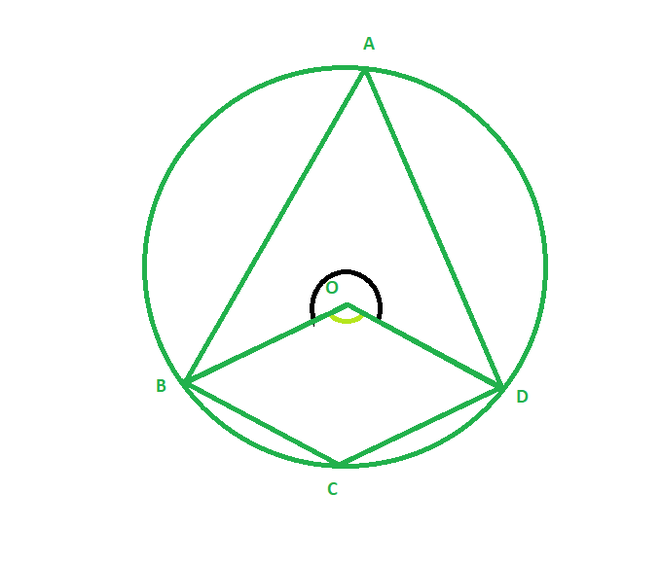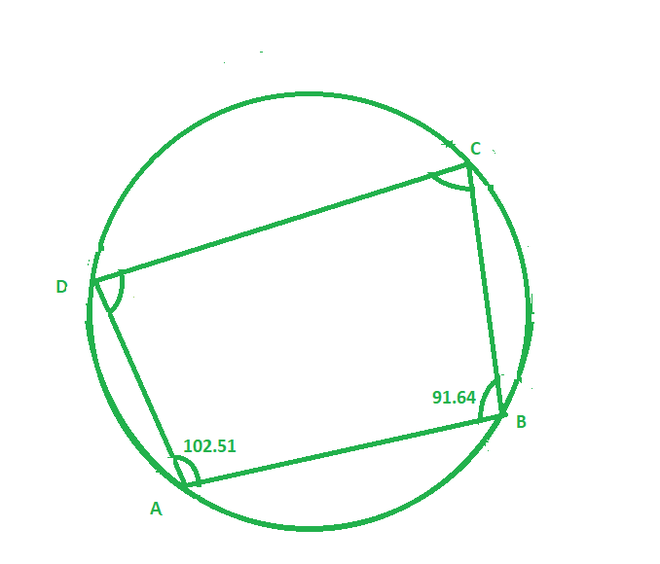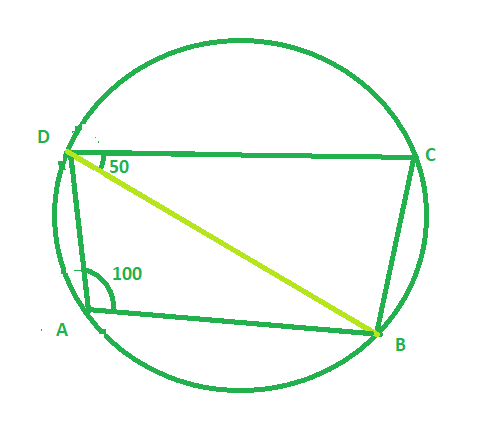# Theorem – The sum of opposite angles of a cyclic quadrilateral is 180° | Class 9 Maths

• Difficulty Level : Medium
• Last Updated : 12 Oct, 2021

In Euclidean geometry, a cyclic quadrilateral or inscribed quadrilateral is a quadrilateral whose vertices all lie on a single circle. This circle is called the circumcircle or circumscribed circle, and the vertices are said to be concyclic. The centre of the circle and its radius are called the circumcenter and the circumradius respectively. Other names for these quadrilaterals are concyclic quadrilateral and chordal quadrilateral, the latter since the sides of the quadrilateral are chords of the circumcircle.

### Theorem Statement: The sum of the opposite angles of a cyclic quadrilateral is 180°.

So according to the theorem statement, in the below figure, we have to prove that

Attention reader! All those who say programming isn't for kids, just haven't met the right mentors yet. Join the  Demo Class for First Step to Coding Coursespecifically designed for students of class 8 to 12.

The students will get to learn more about the world of programming in these free classes which will definitely help them in making a wise career choice in the future.Proof:

Given:

A cyclic quadrilateral ABCD where O is the centre of a circle.

Construction:

Join the line segment OB and OD

Since, The angle subtended by an arc at the centre is double the angle on the circle.

Therefore,

∠BAD = 1/2 (∠BOD)                         – equation 1

Similarly,

∠BCD = 1/2 (reflex of ∠BOD)           – equation 2

By adding equation 1 and 2, we get

∠BAD + ∠BCD = 1/2 (∠BOD + reflex of ∠BOD)

∠BAD + ∠BCD = 1/2 ( 360o)             {Since , ∠BOD + reflex of ∠BOD form complete angle i.e 360 degree}

Similarly,

Hence proved, that the sum of opposite angles of a cyclic quadrilateral is 180°

### Sample Problems on the Given Theorem

Question 1: In the figure given below, ABCD is a cyclic quadrilateral in which ∠CBA = 91.64° and ∠DAB = 102.51° find ∠ADC and ∠DCB?Solution:

By applying theorem,

The sum of opposite angles of a cyclic quadrilateral is 180°

We get,

∠ADC =180o – 91.64o = 88.36 degree

And,

∠DCB + 102.51o = 180o

∠DCB = 180o – 102.51o

∠DCB = 77.49o

Hence ∠ADC = 88.36o and ∠DCB = 77.49o

Question 2: In the figure given below, ABCD is a cyclic quadrilateral in which ∠BAD = 100° and ∠BDC = 50° find ∠DBC?Solution:

Given :

∠BAD = 100°  and ∠CDB = 50o

∠BCD = 180o – 100o = 800

In ∆BCD ,

∠BCD + ∠CDB + ∠DBC = 180o

80o  + 50o + ∠DBC = 180o

∠DBC = 180o  – 130o

∠DBC = 50o

Therefore ∠DBC = 50

My Personal Notes arrow_drop_up